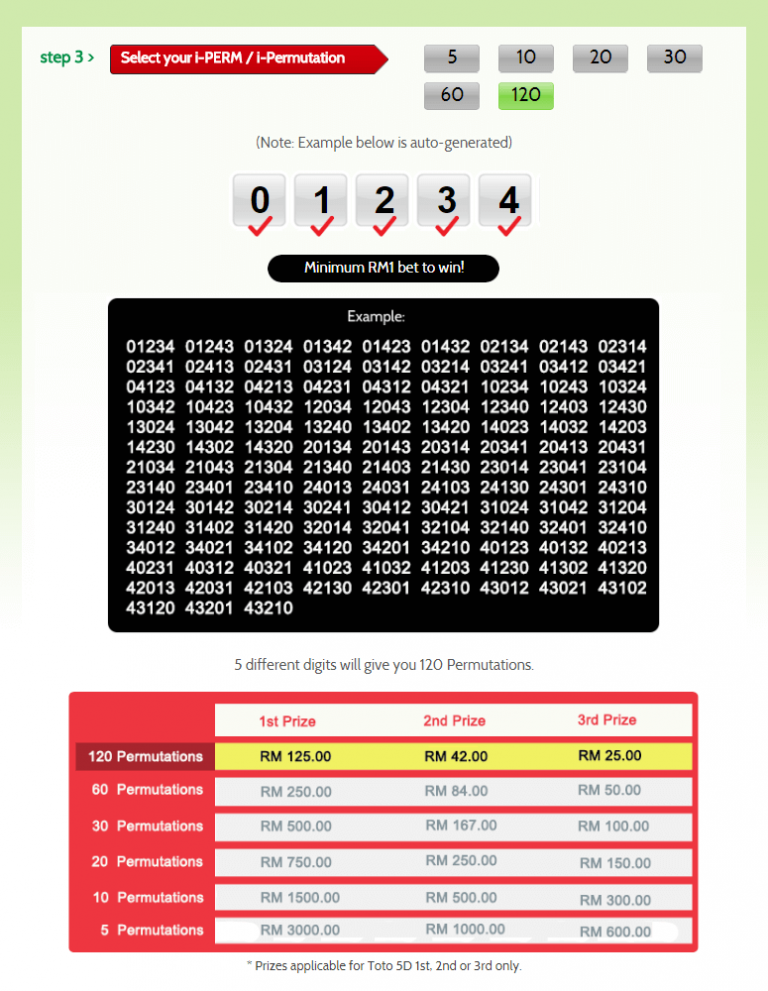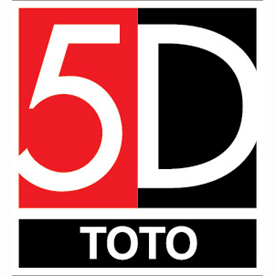Sports TOTO 5D

2 easy steps to winning chances!

1. 5D Standard Game
2. 5D i-Perm / i-Permutation

## 5D Standard Game Play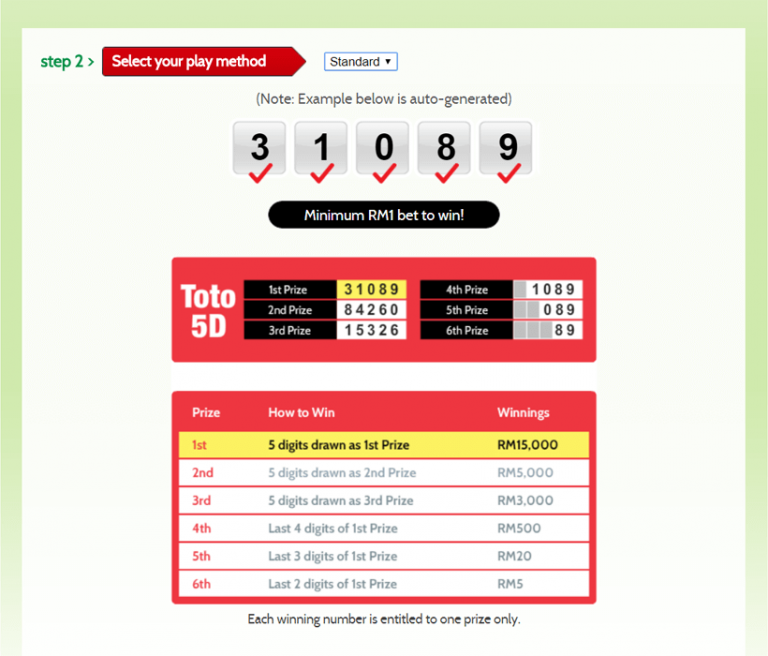5D i-Perm / i-Permutation

i-Perm or Insurance Permutation gives you more chances to win with smaller bets. Each set of permutation costs RM1.00.

Below is an example of a 5-Permutation number that costs RM1.00.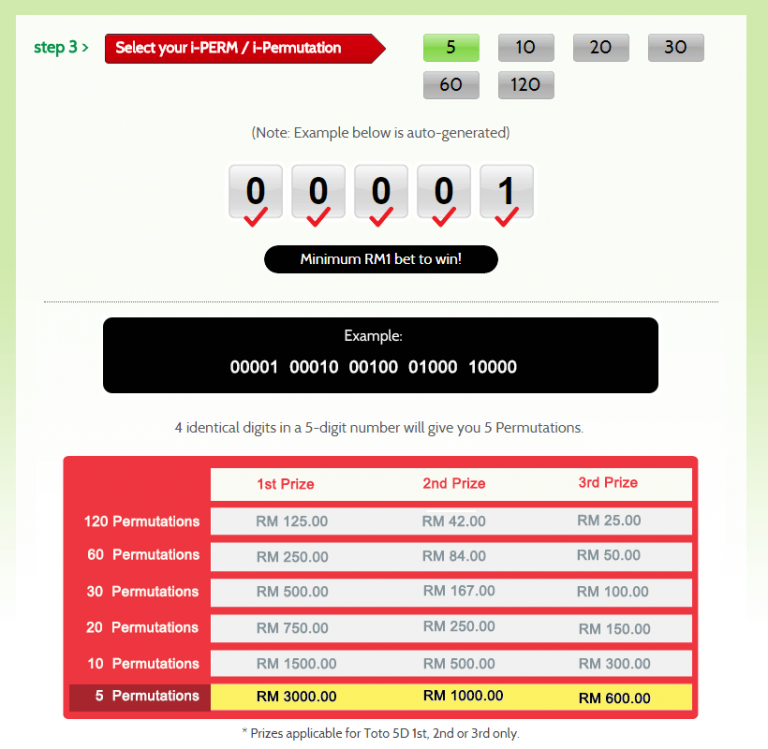Below is an example of a 10-Permutation number that costs RM1.00.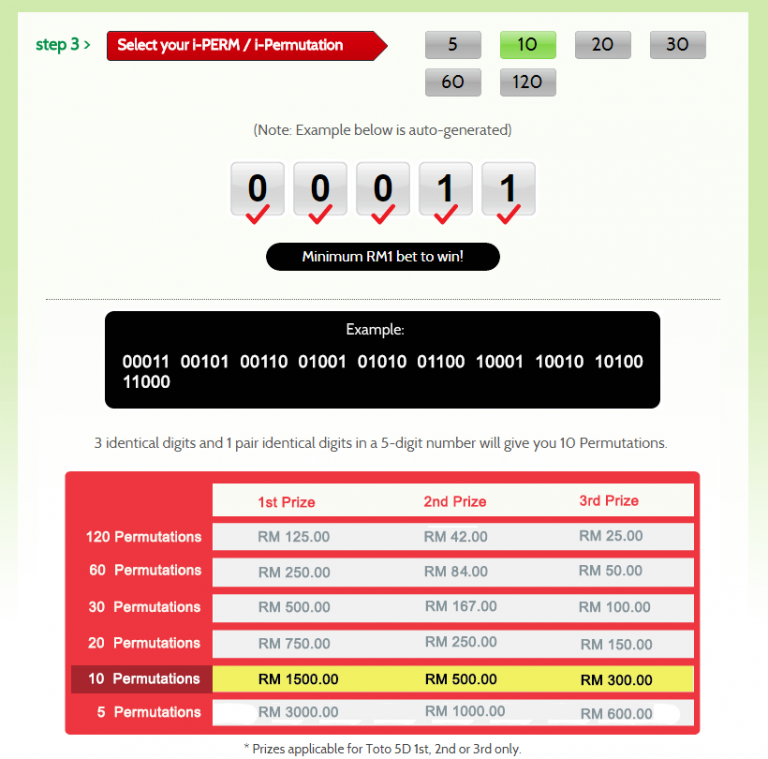Below is an example of a 20-Permutation number that costs RM1.00.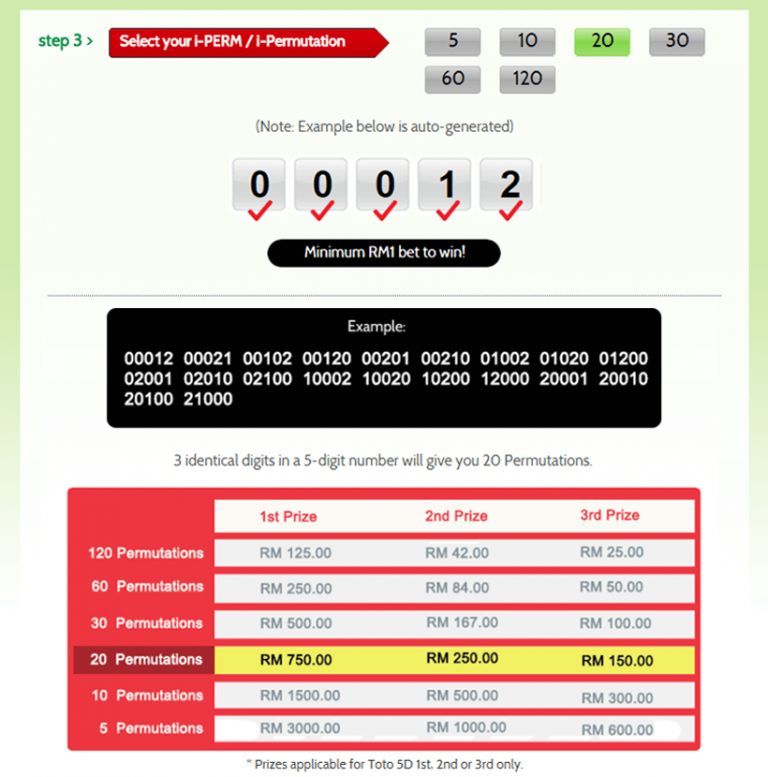Below is an example of a 30-Permutation number that costs RM1.00.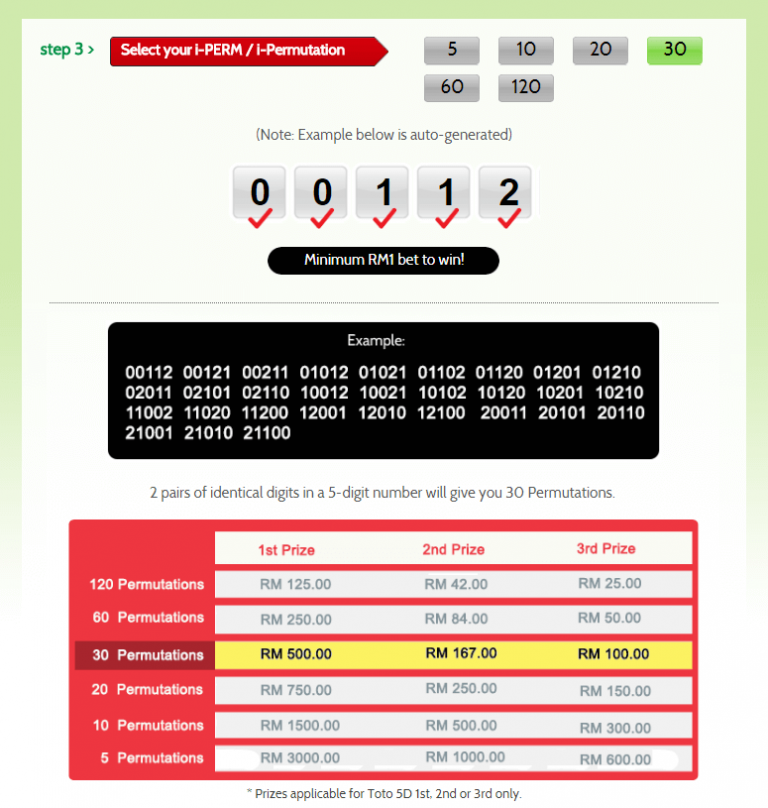Below is an example of a 60-Permutation number that costs RM1.00.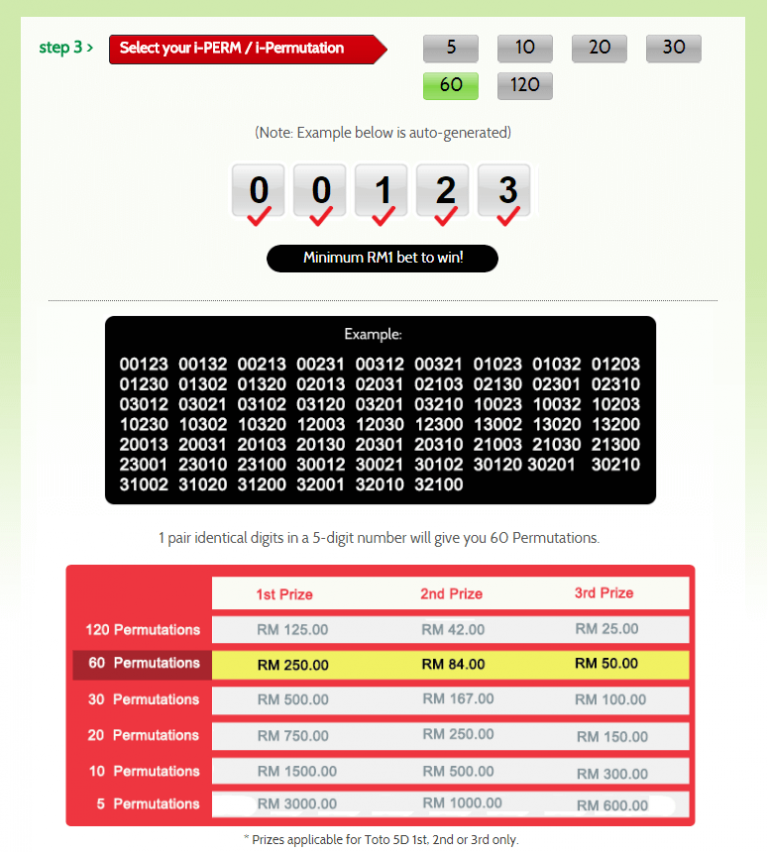Below is an example of a 120-Permutation number that costs RM1.00.